Updating search results...

# 11 Results

View
Selected filters:
• UT.MATH.II.SII.MP.7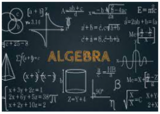Conditional Remix & Share Permitted
CC BY-NC
Rating
0.0 stars

This unit was created as an introductory/review unit for Algebra II to allow for a review of main concepts needed to progress in Algebra II. However, it could also be used in Algebra I to introduce for the first time real numbers;&nbsp;number operations;&nbsp;and equations, expressions, and inequalities.

Subject:
Secondary Mathematics
Material Type:
Homework/Assignment
Lesson
Unit of Study
Author:
Emily
02/22/2022Unrestricted Use
CC BY
Rating
0.0 stars

Subject:
Mathematics
Secondary Mathematics
Material Type:
Activity/Lab
Provider:
Illustrative Mathematics
Provider Set:
Illustrative Mathematics
Author:
Illustrative Mathematics
05/01/2012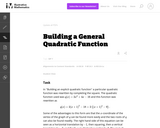Unrestricted Use
CC BY
Rating
0.0 stars

This task is for instructional purposes only and builds on ``Building an explicit quadratic function.''

Subject:
Mathematics
Secondary Mathematics
Material Type:
Activity/Lab
Provider:
Illustrative Mathematics
Provider Set:
Illustrative Mathematics
Author:
Illustrative Mathematics
08/20/2012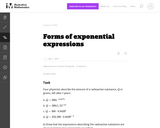Unrestricted Use
CC BY
Rating
0.0 stars

This task contrasts the usefulness of four equivalent expressions. Students first have to confirm that the given expressions for the radioactive substance are equivalent. Then they have to explain the significance of each expression in the context of the situation.

Subject:
Mathematics
Secondary Mathematics
Material Type:
Activity/Lab
Provider:
Illustrative Mathematics
Provider Set:
Illustrative Mathematics
Author:
Illustrative Mathematics
02/03/2013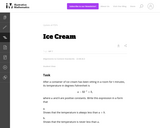Unrestricted Use
CC BY
Rating
0.0 stars

This task illustrates the process of rearranging the terms of an expression to reveal different aspects about the quantity it represents, precisely the language being used in standard A-SSE.B.3.

Subject:
Mathematics
Secondary Mathematics
Material Type:
Activity/Lab
Provider:
Illustrative Mathematics
Provider Set:
Illustrative Mathematics
Author:
Illustrative Mathematics
05/01/2012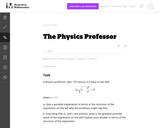Unrestricted Use
CC BY
Rating
0.0 stars

Subject:
Mathematics
Secondary Mathematics
Material Type:
Activity/Lab
Provider:
Illustrative Mathematics
Provider Set:
Illustrative Mathematics
Author:
Illustrative Mathematics
05/01/2012Unrestricted Use
CC BY
Rating
0.0 stars

This task compares the usefulness of different forms of a quadratic expression. Students have to choose which form most easily provides information about the maximum value, the zeros and the vertical intercept of a quadratic expression in the context of a real world situation. Rather than just manipulating one form into the other, students can make sense out of the structure of the expressions.

Subject:
Mathematics
Secondary Mathematics
Material Type:
Activity/Lab
Provider:
Illustrative Mathematics
Provider Set:
Illustrative Mathematics
Author:
Illustrative Mathematics
05/01/2012Unrestricted Use
CC BY
Rating
0.0 stars

This question provides students with an opportunity to see expressions as constructed out of a sequence of operations: first taking the square root of n, then dividing the result of that operation into s. Students studying statistics encounter the expression in this question as the standard deviation of a sampling distribution with samples of size n when the distribution from which the sample is taken has standard deviation s.

Subject:
Mathematics
Secondary Mathematics
Material Type:
Activity/Lab
Provider:
Illustrative Mathematics
Provider Set:
Illustrative Mathematics
Author:
Illustrative Mathematics
05/01/2012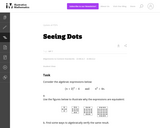Unrestricted Use
CC BY
Rating
0.0 stars

The purpose of this task is to identify the structure in the two algebraic expressions by interpreting them in terms of a geometric context. Students will have likely seen this type of process before, so the principal source of challenge in this task is to encourage a multitude and variety of approaches, both in terms of the geometric argument and in terms of the algebraic manipulation.

Subject:
Mathematics
Secondary Mathematics
Material Type:
Activity/Lab
Provider:
Illustrative Mathematics
Provider Set:
Illustrative Mathematics
Author:
Illustrative Mathematics
05/17/2013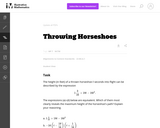Unrestricted Use
CC BY
Rating
0.0 stars

This task illustrates A-SSE.1a because it requires students to identify expressions as sums or products and interpret each summand or factor.

Subject:
Mathematics
Secondary Mathematics
Material Type:
Activity/Lab
Provider:
Illustrative Mathematics
Provider Set:
Illustrative Mathematics
Author:
Illustrative Mathematics